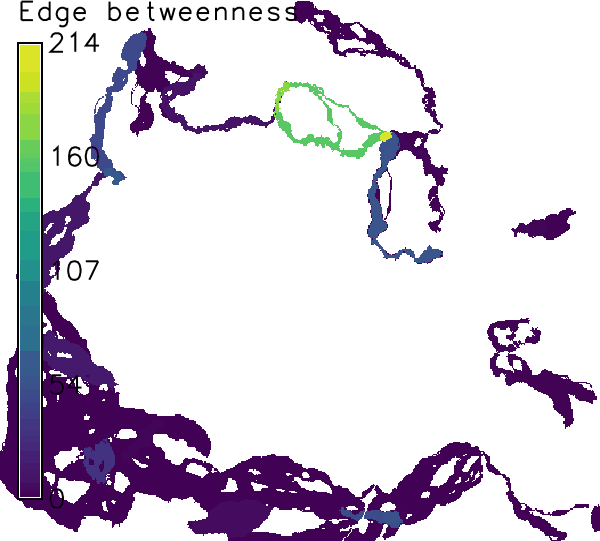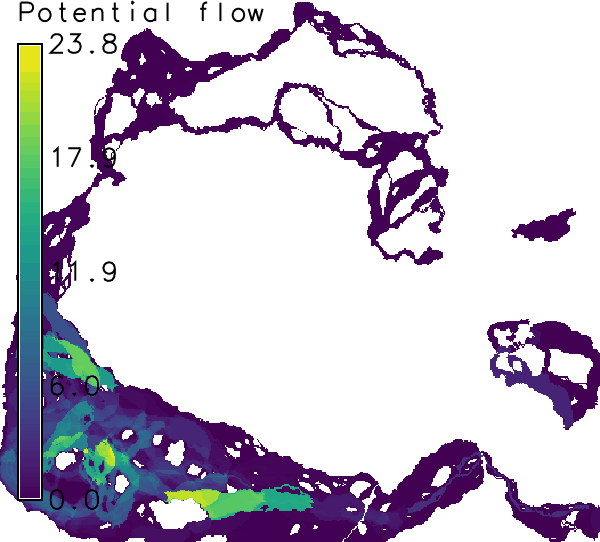## NAME

r.connectivity.corridors - Compute corridors between habitat patches of an input-layer based on (cost) distance raster maps

## KEYWORDS

raster, vector, corridor, cost distance, least cost paths

## SYNOPSIS

r.connectivity.corridors
r.connectivity.corridors --help
r.connectivity.corridors [-sdr] input=Result from r.connectivity.network containing edge measures layer=string [weights=Column names[,Column names,...]] [where=where (SQL statement)] suffix=string [corridor_tolerance=float] [cores=integer] [--help] [--verbose] [--quiet] [--ui]

### Flags:

-s
Show edges selected by where-clause and exit
-d
Assign distance values to corridors instead of connection ids and weights
-r
Recalculate already computed corridors (default is only weight and summarize already existing corridor maps)
--help
Print usage summary
--verbose
Verbose module output
--quiet
Quiet module output
--ui
Force launching GUI dialog

### Parameters:

input=Result from r.connectivity.network containing edge measures [required]
Name of input vector map
Name of the table containing edge measures from r.connectivity.network
layer=string [required]
Layer number or name
layer containing patch geometries
Default: 1
weights=Column names[,Column names,...]
Column names separated by commas
where=where (SQL statement)
WHERE conditions of SQL statement without 'where' keyword
where conditions of an SQL statement without 'where' keyword. (example: cf_mst_ud = 1 or cf_eb_ud > 100)
suffix=string [required]
Output suffix for corridor summary result
corridor_tolerance=float
Tolerance for deviation from (cost) distance within corridors (in %)
Default: 0.0
cores=integer
Default: 1

## DESCRIPTION

r.connectivity.corridors is the 3rd tool of the r.connectivity.* toolset.

r.connectivity.corridors loops through the user-selected edges (where option) of the edge-measure output vector map from r.connectivity.network and computes the respective corridor(s).

Corridors are defined as all pixels that have a lower average cost distance from a pair of patches than the average cost distance between that pair. The user can add a tolerance for allowed deviation from cost distance within corridors in %, using the corridor_tolerance option.

r.connectivity.corridors can account for the importance of the corridors for the entire network by weighting them with regards to one or more network measures from r.connectivity.network, using the weights option.

Thus, r.connectivity.corridors produces two types of output, that are named according to a user defined prefix and suffix:
1. a set of individual corridor raster maps (named: prefix_corridor_edge_id_weight) and
2. a summary over all computed corridors (named: prefix_corridor_edge_id_weight_sum_suffix).

Finally, all individual corridors are being put together using r.series. In this step, the values of the cells in all corridor maps are summed up, which indicates the importance of an area (raster cell) for the network of the given patches (either the number of corridors a cell is part of, or other graph-theoretical measures for corridor importance).

## NOTES

Corridors are only computed for an undirected graph.

## EXAMPLE

The following example is continued from and based on the example in r.connectivity.network!

In this example two alternative (or to some extent complementary) sets of corridors are calculated:

### Corridor set 1:

For the first set, corridors are only computed for the minimum spanning tree (in literature sometimes called the backbone of an ecological network). This is specified in the "where"-parameter. Edge betweenness values are used as weights, which represent the centrality of a connection (edge) in a network (and is therewith a measure of importance). The output is a set of corridor raster maps (named: "prefix" _corridor_ "edge_id" _ "weight") and a summary over all computed corridors (named: "prefix" _corridor_ "edge_id" _ "weight" _sum_ "suffix").
```r.connectivity.corridors input=hws_connectivity_edge_measures layer=1 \
weights=cd_eb_ud suffix=mst corridor_tolerance=0.05 where="cf_mst_ud>0" \
cores=1
```Figure: Corridors computed for connections on the minimum spanning tree weighted by edge betweenness in the example above.

### Corridor set 2:

For the second set, corridors are computed for all edges in the graph (network). This takes a little more time. Corridors are then weighted by the modeled, undirected (= in oth directions) flow of propagules ("cf_u").
```r.connectivity.corridors input=hws_connectivity_edge_measures layer=1 \
weights=cf_u suffix=all corridor_tolerance=0.05 \
cores=1
```Figure: Corridors computed for all connections, weighted by potential flow of propagules.
Users with a multi-processor computer (e.g. dual-core) may speed up corridor processing by setting cores > 1 r.connectivity.corridors.
Another means for speeding up processing would be to use integer weights (e.g. by converting edge betweenness to integer after multiplying with 10 to the power of the relevant number of digits). Then r.reclass is used in the script instead of r.recode.

When all analysis is finished some cleanup may be appropriate, in order to save disk space:
```# Add the f-lag (-f) to the following two commands if you are sure you
# want to delete all intermediate maps from r.connectivity.*.
g.remove type=raster pattern=hws_connectivity_corridor_*
g.remove type=raster pattern=hws_connectivity_patch_*
```

r.connectivity.distance, r.connectivity.network

## AUTHOR

Stefan Blumentrath, Norwegian Institute for Nature Research (NINA)

## SOURCE CODE

Available at: r.connectivity.corridors source code (history)

Latest change: Thu Feb 3 09:32:35 2022 in commit: f17c792f5de56c64ecfbe63ec315307872cf9d5c

© 2003-2022 GRASS Development Team, GRASS GIS 8.0.3dev Reference Manual